Linear system

A family of effective linearly equivalent divisors (cf. Divisor (algebraic geometry)) on an algebraic variety, parametrized by projective space.

Letbe a non-singular algebraic variety over a field,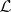an invertible sheaf on,the space of global sections of, and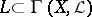a finite-dimensional subspace. If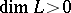, then the divisors determined by zero sections ofare linearly equivalent and effective. A linear system is the projective space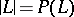of one-dimensional subspaces ofthat parametrizes these divisors. If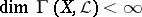, then the linear system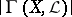is said to be complete; it is denoted by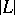.

Letbe a basis of. It defines a rational mapping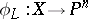by the formula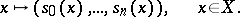One usually says that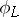is defined by the linear system. The imagedoes not lie in any hyperplane of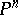(see ). Conversely, every rational mapping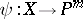having this property is defined by some linear system.

A fixed component of a linear systemis an effective divisor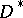onsuch thatfor any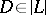, where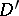is an effective divisor. Whenruns through, the divisorsform a linear system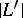of the same dimension as. The mappingcoincides with. Therefore, in consideringone may assume thatdoes not have fixed components. In this caseis not defined exactly on the basic set of.

Contents

Examples.

1) Let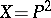and,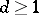; then the sections of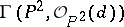can be identified with forms of degreeon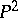, and the complete linear systemcan be identified with the set of all curves of order.

2) The standard quadratic transformation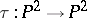(see Cremona transformation) is defined by the linear system of conics passing through the points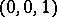,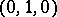,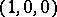.

3) The Geiser involution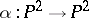is defined by the linear system of curves of order 8 passing with multiplicity 3 through 7 points in general position (cf. Point in general position).

4) The Bertini involution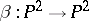is defined by the linear system of curves of order 17 passing with multiplicity 6 through 8 points in general position.